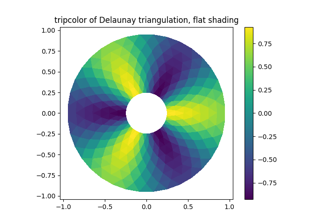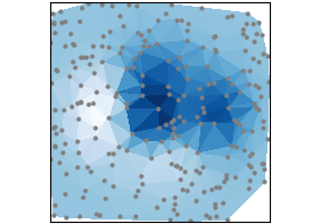# matplotlib.axes.Axes.tripcolor#

Axes.tripcolor(*args, alpha=1.0, norm=None, cmap=None, vmin=None, vmax=None, shading='flat', facecolors=None, **kwargs)[source]#

Create a pseudocolor plot of an unstructured triangular grid.

Call signatures:

```tripcolor(triangulation, C, *, ...)
```

The triangular grid can be specified either by passing a `Triangulation` object as the first parameter, or by passing the points x, y and optionally the triangles and a mask. See `Triangulation` for an explanation of these parameters.

It is possible to pass the triangles positionally, i.e. `tripcolor(x, y, triangles, C, ...)`. However, this is discouraged. For more clarity, pass triangles via keyword argument.

If neither of triangulation or triangles are given, the triangulation is calculated on the fly. In this case, it does not make sense to provide colors at the triangle faces via C or facecolors because there are multiple possible triangulations for a group of points and you don't know which triangles will be constructed.

Parameters:
triangulation`Triangulation`

Parameters defining the triangular grid. See `Triangulation`. This is mutually exclusive with specifying triangulation.

Carray-like

The color values, either for the points or for the triangles. Which one is automatically inferred from the length of C, i.e. does it match the number of points or the number of triangles. If there are the same number of points and triangles in the triangulation it is assumed that color values are defined at points; to force the use of color values at triangles use the keyword argument `facecolors=C` instead of just `C`. This parameter is position-only.

facecolorsarray-like, optional

Can be used alternatively to C to specify colors at the triangle faces. This parameter takes precedence over C.

If 'flat' and the color values C are defined at points, the color values used for each triangle are from the mean C of the triangle's three points. If shading is 'gouraud' then color values must be defined at points.

other_parameters

All other parameters are the same as for `pcolor`.

## Examples using `matplotlib.axes.Axes.tripcolor`#Tripcolor Demo

Tripcolor Demotripcolor(x, y, z)

tripcolor(x, y, z)